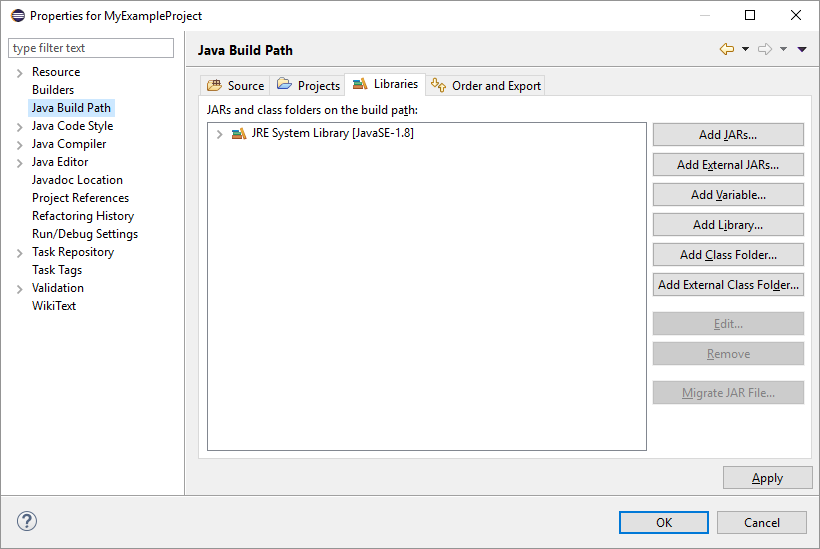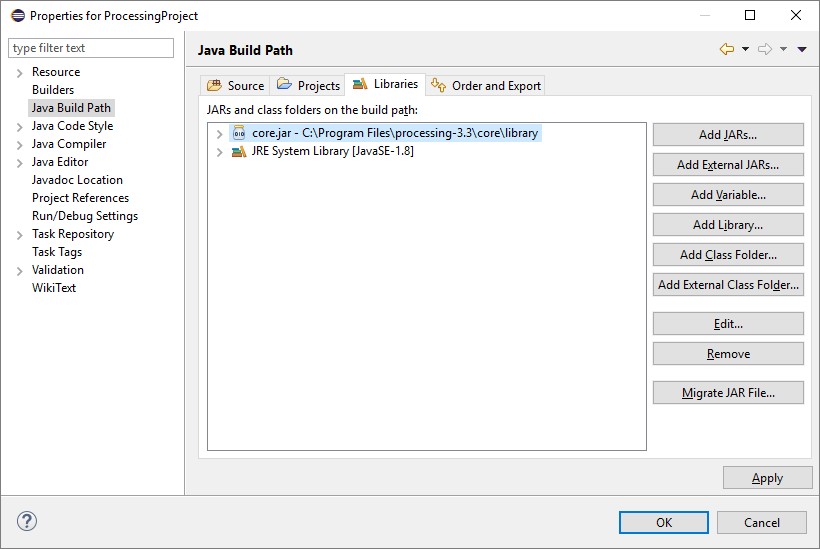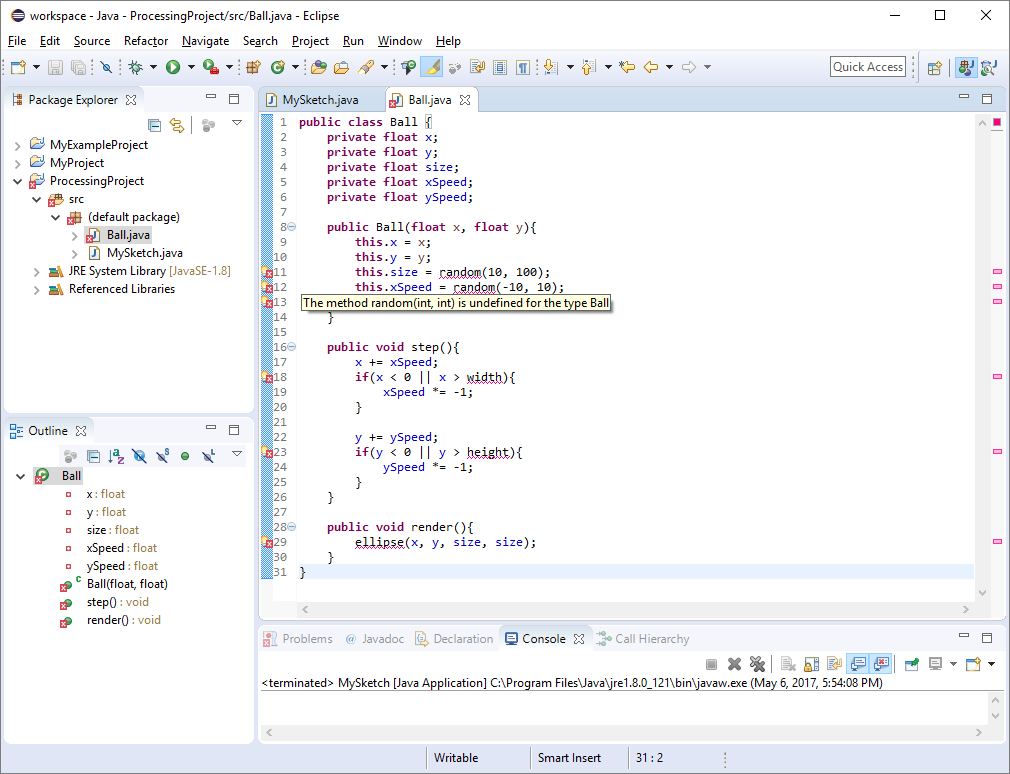# Processing in Java

tutorial java processing

At this point you’ve probably used Processing through the Processing editor, which lets you write Processing code and automatically run it. You’ve also learned how to use Java libraries, which allow you to use classes and functions written by other people in your Java code. This tutorial combines those ideas and shows you how to use Processing as a Java library.

## Processing is Java

So far, we’ve used Processing through the Processing editor, which lets us write Processing code that the editor then runs when you press the play button. But behind the scenes, when you press the play button, the Processing editor is translating your Processing code into Java code, compiling it, and running it.

For example, we might write Processing code that looks like this:

``````void setup(){
size(500, 500);
}

void draw(){
background(64);
ellipse(mouseX, mouseY, 20, 20);
}
``````

When we run this code, Processing is secretly turning this into Java code that looks like this:

``````import processing.core.PApplet;

public class MySketch extends PApplet {

public void settings() {
size(500, 500);
}

public void draw(){
background(64);
ellipse(mouseX, mouseY, 20, 20);
}

public static void main(String[] passedArgs) {
String[] appletArgs = new String[] { "MySketch" };
PApplet.main(appletArgs);
}
}
``````

You can see this yourself by exporting a Processing sketch as an application and then looking at the `.java` file it generates.

This works because Processing is written in Java. So the Processing editor can take your Processing code and make a few minor adjustments, such as putting it inside a class inside a `.java` file, to create valid Java code. Then it compiles and runs that Java code, exactly how we’ve been compiling and running our Java code in these tutorials.

And because Processing is written in Java, we can do the exact same thing from our Java code. This lets us write Java code that accesses Processing’s functionality directly, without going through the Processing editor. This is useful for writing more complicated Processing sketches, or for writing a library that you can then use in Processing.

This tutorial uses Eclipse, but you can also do this from the command line.

First, create a new project in Eclipse (check out the Projects section of the Eclipse tutorial if you need a reminder).

To use Processing in our Java code, we have to include Processing on our classpath. To set your project’s classpath, right-click your project, and then click the `Properties` option. This brings up a dialog with all the settings you can specify for your project. Click the `Java Build Path` menu, and then the `Libraries` tab, which takes you to this screen:On this screen, click the `Add External JARs...` button, and then navigate to your Processing directory. I put mine in my `Program Files` directory, so I navigate to `C:/Program Files/processing-3.3`. That directory contains a `core` directory, which then contains a `libraries` directory, which finally contains a bunch of `.jar` files. These are the `.jar` files that make up the Processing library!

For now we only need the `core.jar` file, so our classpath should look like this:Click `OK` in that window. Now you’re ready to use Processing in your Java code!

## Extending the `PApplet` Class

Remember from the inheritance tutorial that we can extend a class to create a child class that is a more specific version of a parent class- like a square is a more specific version of a rectangle, which is a more specific version of a shape. This allows code to deal with a parent class without worrying about specific child classes.

In Procesing, every sketch is a `PApplet`, so if you want to create a Processing sketch, then the first thing you need to do is create a class that extends the `PApplet` class.

``````import processing.core.PApplet;

public class MySketch extends PApplet{

}
``````

Remember that extending a class sets up an is-a relationship, so an instance of our `MySketch` class is an instance of the `PApplet` class. That means we can pass an instance of this class to anything that works with an instance of the `PApplet` class. Specifically, we can pass instances of this into Processing!

``````import processing.core.PApplet;

public class MySketch extends PApplet{

public static void main(String[] args){
String[] processingArgs = {"MySketch"};
MySketch mySketch = new MySketch();
PApplet.runSketch(processingArgs, mySketch);
}
}
``````

This code calls the `PApplet.runSketch()` function, which expects two parameters: an array of arguments (by default, you only need one argument, the name of the sketch), and an instance of `PApplet` that contains your sketch code. the `runSketch()` function then displays a window and runs your sketch inside that window. If you run this code, you should see something like this:So far that’s not very exciting, because we haven’t told our sketch to do anything yet!

## Overriding Processing Functions

Remember that we can override parent class functions in a child class to specify our own behavior. For example, in the Swing tutorial we extended the `JPanel` class and overrided the `paintComponent()` function to perform custom painting.

When using Processing as a Java library, we want to do something similar: we’ve extended the `PApplet` class, and now we want to override various functions to change their behavior- these are the Processing functions you’re used to, like `draw()` and `mousePressed()`.

``````import processing.core.PApplet;

public class MySketch extends PApplet{

public void settings(){
size(500, 500);
}

public void draw(){
ellipse(mouseX, mouseY, 50, 50);
}

public void mousePressed(){
background(64);
}

public static void main(String[] args){
String[] processingArgs = {"MySketch"};
MySketch mySketch = new MySketch();
PApplet.runSketch(processingArgs, mySketch);
}
}
``````

Most of this should look pretty familiar, but there are a few things to note:

• The functions are `public`.
• We use `settings()` instead of `setup()`.
• We don’t have access to the `color` type.

Notice that we have access to the `mouseX` and `mouseY` variables, along with all of the other variables we’ve used in Processing. That’s because they’re defined in the `PApplet` class, and since our class extends `PApplet`, our class automatically has access to all of those variables.

Also notice that Processing is still handling a lot of stuff for us: creating a window, calling `draw()` 60 times per second, updating the `mouseX` and `mouseY` variables, and calling the `mousePressed()` function.

This means we get the best of both worlds: we can use Eclipse to create projects that consist of a bunch of files, but we can still use Processing to give us an easy way to create a visualization.

## Command Line

Just to show that you can do all of this from the command line, here are the commands for compiling and running the above class:

``````> javac -cp ".;C:\Program Files\processing-3.3\core\library\core.jar" MySketch.java
> java -cp ".;C:\Program Files\processing-3.3\core\library\core.jar" MySketch
``````

## Calling Processing Functions From Non-Sketch Classes

Let’s say we have a `Ball.java` class that looks like this:

``````public class Ball {
private float x;
private float y;
private float size;
private float xSpeed;
private float ySpeed;

public Ball(float x, float y){
this.x = x;
this.y = y;
this.size = random(10, 100);
this.xSpeed = random(-10, 10);
this.ySpeed = random(-10, 10);
}

public void step(){
x += xSpeed;
if(x < 0 || x > width){
xSpeed *= -1;
}

y += ySpeed;
if(y < 0 || y > height){
ySpeed *= -1;
}
}

public void render(){
ellipse(x, y, size, size);
}
}
``````

This class encapsulates variables required to make a ball bounce around on the screen. It uses Processing’s `width` and `height` variables, as well as the `random()` and `ellipse()` functions. This would work in the Processing editor, but in Eclipse (or in the command line), we’ll get a bunch of compiler errors:These errors are telling us that Java can’t reach Processing’s variables and functions from our `Ball` class. And that makes sense, becuase Processing’s variables and functions are defined inside the `PApplet` class.

In other words, variables like `width` and `height` and functions like `ellipse()` belong to a particular sketch, and a ball is not a sketch. So what we need to do is pass a reference to our sketch into our `Ball` class, and then use the sketch variable to access Processing’s variables and functions. That might sound complicated, but it looks like this:

``````import processing.core.PApplet;

public class Ball {

private PApplet sketch;

private float x;
private float y;
private float size;
private float xSpeed;
private float ySpeed;

public Ball(PApplet sketch, float x, float y){
this.sketch = sketch;
this.x = x;
this.y = y;
this.size = sketch.random(10, 100);
this.xSpeed = sketch.random(-10, 10);
this.ySpeed = sketch.random(-10, 10);
}

public void step(){
x += xSpeed;
if(x < 0 || x > sketch.width){
xSpeed *= -1;
}

y += ySpeed;
if(y < 0 || y > sketch.height){
ySpeed *= -1;
}
}

public void render(){
sketch.ellipse(x, y, size, size);
}
}
``````

Now this code accepts a `PApplet` instance as an argument to its constructor, which it saves in an instance variable. This gives use access to a particular sketch, so whenever we want to use a Processing variable or function, we use the `sketch` variable.

We can now use this class in our main sketch class:

``````import java.util.ArrayList;
import processing.core.PApplet;

public class MySketch extends PApplet{

private ArrayList<Ball> balls = new ArrayList<>();

public void settings(){
size(500, 500);
}

public void draw(){
background(64);
for(Ball b : balls){
b.step();
b.render();
}
}

public void mouseDragged(){
}

public static void main(String[] args){
String[] processingArgs = {"MySketch"};
MySketch mySketch = new MySketch();
PApplet.runSketch(processingArgs, mySketch);
}
}
``````

This code uses the `this` keyword to pass a reference to the current instance into the `Ball` constructor. So now when we call `sketch.ellipse()` from the `Ball` class, it draws to our sketch.

## Creating Processing Libraries

You can use the above approach of accepting a `PApplet` argument into your code to create a library that you can then use in a Processing sketch in the Processing editor.

Then you would export a `.jar` file that contains your code. Make sure your classes are in packages: classes inside `.jar` files must be inside a package to be visible to other classes inside a package. (The alternative is to not use packages at all, which is generally a bad idea.)

Now that you have a `.jar` file, you can drag it directly onto the Processing editor, or you can package it up as a “real” Processing library for other people to use. See the Processing wiki for more info.

## Homework

• Create a Swing GUI that contains buttons that control a sketch window. For example, you could show arrow buttons that move a ball in the Processing window.
• Create a Java application that uses the Twitter4J library along Processing as a Java library to create a Twitter bot!
• Create a Java application that lets the user choose from all of the sketches you’ve created so far.